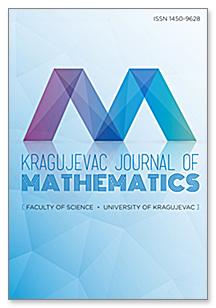eLibrary of Mathematical Instituteof the Serbian Academy of Sciences and Arts> Home / All Journals / Journal /Kragujevac Journal of MathematicsPublisher: Prirodno-matematički fakultet Kragujevac, KragujevacISSN: 1450-9628Issue: 46_2Date: 2022Journal Homepage

 Paranormed Riesz Difference Sequence Spaces of Fractional Order 175 - 191 Taja Yaying
AbstractKeywords: Riesz difference sequence spaces; difference operator $\Delta^{(\alpha)}$; Schauder basis; $\alpha$-; $\beta$-; $\gamma$- duals; matrix transformationMSC: 46A45 46A35; 46B45
 General Classes of Shrinkage Estimators for the Multivariate Normal Mean with Unknown Variance: Minimaxity and Limit of Risks Ratios 193 - 213 Abdelkader Benkhaled and Abdenour Hamdaoui
AbstractKeywords: James-Stein estimator; multivariate Gaussian random variable; non-central chi-square distribution; quadratic risk; shrinkage estimatorMSC: 62F12 62C20
 On $(m,h_1,h_2)$-G-Convex Dominated Stochastic Processes 215 - 227 Jorge Eliecer Hernández Hernández
AbstractKeywords: $(m;h_1;h_2)$-convexity; dominated convexity; mean square integral inequalities; stochastic processesMSC: 52A01 26D15; 60E15
 $\alpha\beta$-Weighted $d_g$-Statistical Convergence in Probability 229 - 239 Mandobi Banerjee
AbstractKeywords: $\alpha\beta$-weighted $d_g$-statistical convergence in probability; $\alpha\beta$-weighted $d_g$-strongly Cesàro convergence in probability; $g$-weighted $S_\alpha\beta$-convergence in probability; $g$-weighted $N_\alpha\beta$-convergence in probabilityMSC: 40A35 40G15; 60B10
 Some Remarks on Various Schur Convexity 241 - 257 Farzaneh Gorjizadeh and Noha Eftekhari
AbstractKeywords: Schur convex; Schur geometrically convex; Schur harmonic convexMSC: 26B25 05E05; 26D20
 On Rapid Equivalence and Translational Rapid Equivalence 259 - 265 Valentina Timotić, Dragan Djurčić and Mališa R. Žižović
AbstractKeywords: Rapid equivalence; the class $R_\infty;s$; selection principlesMSC: 26A12 40A05; 91A05
 Well-Posedness and General Decay of Solutions for the Heat Equation with a Time Varying Delay Term 267 - 282 Abdelkader Braik, Abderrahmane Beniani and Yamina Miloudi
AbstractKeywords: Heat equation; time varying delay; energy decay; Lyapunov functional; global existence; viscoelastic termMSC: 35L05; 35L15 35L70; 35B05
 Riesz Lacunary Sequence Spaces of Fractional Difference Operator 283 - 294 Kuldip Raj, Kavita Saini and Neeru Sawhney
AbstractKeywords: Fractional difference operator; gamma function; modulus function; Riesz mean; lacunary sequencesMSC: 46A45; 46A35; 40A05
 Generalized Averaged Gaussian Formulas for Certain Weight Functions 295 - 305 Rada M. Mutavdžić
AbstractKeywords: Gauss quadrature; Gauss-Kronrod quadrature; averaged Gaussian formulas; truncations of averaged Gaussian formulasMSC: 65D30 65D32
 $(m,n)$-Hyperfilters in Ordered Semihypergroups 307 - 315 Ahsan Mahboob and Noor Mohammad Khan
AbstractKeywords: Ordered semihypergroups; left-$m$-hyperfilters; right-$n$-hyperfilters; $(m;n)$-hyperfiltersMSC: 20N20
Article page: 12>>

 Remote Address: 34.204.166.236 • Server: elib.mi.sanu.ac.rsHTTP User Agent: CCBot/2.0 (https://commoncrawl.org/faq/)